# Increase the conversion rate of telemarketing campaigns in a bank using Artificial IntelligenceCustomer targeting consists of identifying those persons that are more prone to a specific product or service. This example aims to predict whether bank clients will subscribe to a long-term deposit and which will not. The data set used here is related to the direct marketing campaigns of a Portuguese bank institution.

### Contents:

This example is solved with Neural Designer. To follow it step by step, you can use the free trial.

## 1. Application type

The variable to be predicted is binary (buy or not buy). Thus, this is a classification project. The goal here is to model the probability of buying as a function of the customer features.

## 2. Data set

In general, a data set contains the following concepts:
• Data source.
• Variables.
• Instances.
• Missing values.
The data file bank_marketing.csv contains the information used to create the model. It consists of 1522 rows and 19 columns. Each row represents a different customer, while each column represents a distinct feature for each customer. The variables are:
• age: Age.
• married: Marital status.
• single: Marital status.
• divorced: Marital status.
• education: Type of education (primary, secondary, tertiary).
• default: Takes value 1 if the client has a credit in default and 0 in other cases.
• balance: Account balance.
• housing: Takes value 1 if the client has a housing loan and 0 in other cases.
• loan: Takes value 1 if the client has a personal loan and 0 in other cases.
• contact: Contact communication type (cellular, telephone).
• day: Last contact day of the week.
• month: Last contact month of the year.
• campaign: Number of contacts performed during this campaign and for this client.
• pdays: Number of days that passed by after the client was last contacted from a previous campaign.
• previous: Number of contacts performed before this campaign and for this client.
• poutcome: Outcome of the previous marketing campaign.
• conversion: Takes value 1 if the client has subscribed to a term deposit and 0 in other cases, used as the target.
There are 1522 instances in the data set. 60% are used for training, 20% for selection, and 20% for testing. We can calculate the data distribution to see the percentage of instances for each class.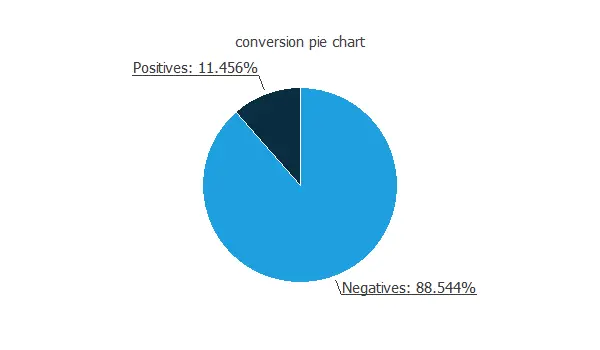As expected, the number of calls without conversion is much greater than the number of calls with conversion. We can also calculate the inputs-targets correlations between the conversion rate and all the customer features to see which variables might influence the buying process.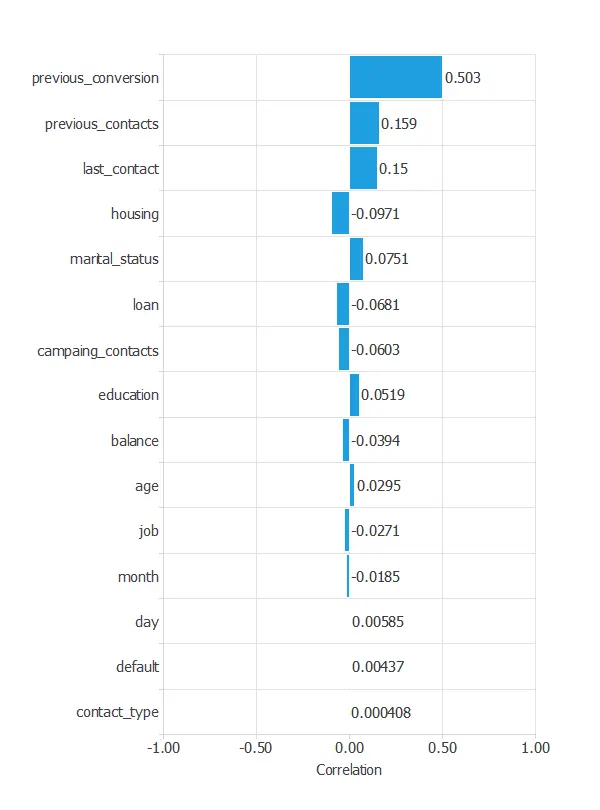## 3. Neural network

The second step is to configure the neural network parameters. For classification problems, it is composed of:
• Scaling layer.
• Perceptron layers.
• Probabilistic layer.
The following figure is a graphical representation of the neural network used for this problem.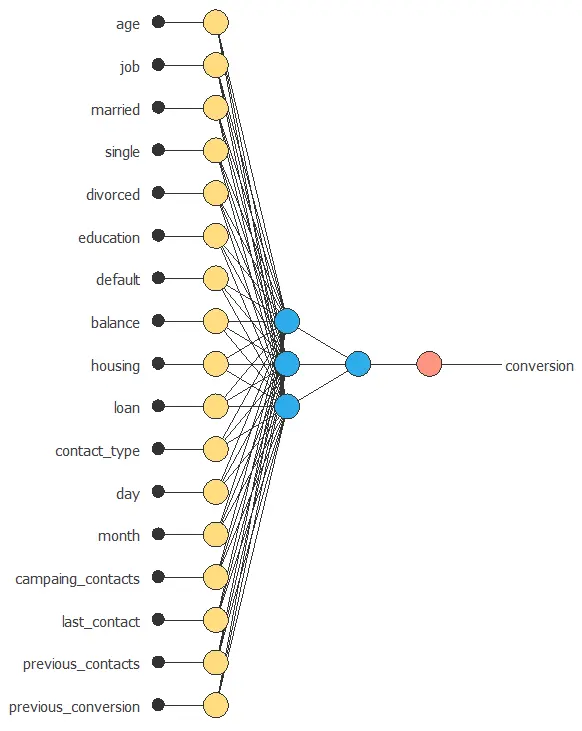## 4. Training strategy

The fourth step is to configure the training strategy, which is composed of two concepts:
• A loss index.
• An optimization algorithm.
The loss index chosen is the weighted squared error with L2 regularization. The optimization algorithm is applied to the neural network to get the minimum loss. The chosen algorithm here is the quasi-Newton method. We leave the default training parameters, stopping criteria, and training history settings. The following chart shows how the training and selection errors decrease with the epochs during the training process. The final values are training error = 0.821 WSE and selection error = 0.889 WSE, respectively.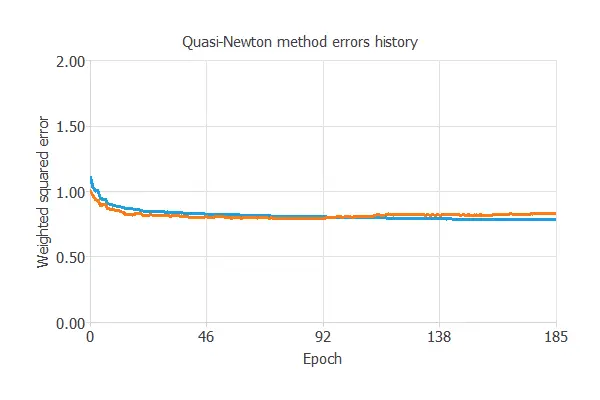## 5. Model selection

The objective of model selection is to find a network architecture with the best generalization properties, that is, that which minimizes the error on the selected instances of the data set. More specifically, we want to find a neural network with a selection error of less than 0.889 WSE, which is the value that we have achieved so far. Order selection algorithms train several network architectures with a different number of neurons and select that with the smallest selection error. The incremental order method starts with a small number of neurons and increases the complexity at each iteration. The following chart shows the training error (blue) and the selection error (orange) as a function of the number of neurons.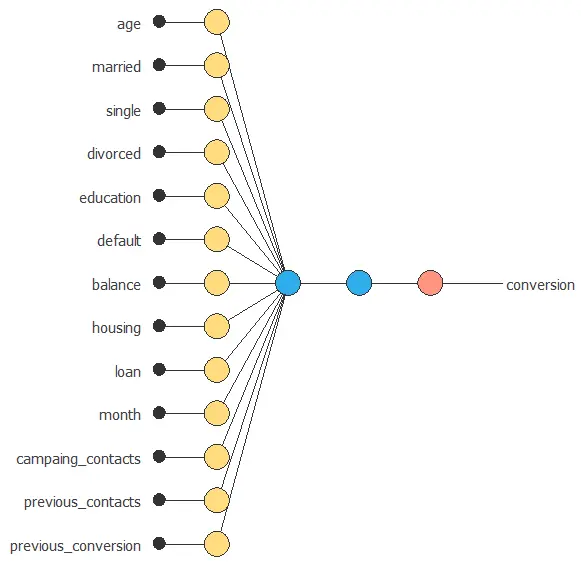## 6. Testing analysis

The objective of the testing analysis is to evaluate the generalization performance of the neural network. The standard way to do this is to compare the neural network outputs against data that it has never seen before, the testing instances. A commonly used method to test a neural network is the ROC curve.One of the parameters obtained from this chart is the area under the curve (AUC). The closer to 1 is the area under the curve, the better is the classifier. In this case, the area under the curve takes a high value: AUC = 0.80. The binary classification tests provide us with useful information for testing the performance of a binary classification problem:
• Classification accuracy: 79.4% (ratio of correctly classified samples).
• Error rate: 20.6% (ratio of misclassified samples).
• Sensitivity: 80.4% (percentage of actual positive classified as positive).
• Specificity: 79.3% (percentage of actual negative classified as negative).
The classification accuracy takes a high value, which means that the prediction is suitable for many cases. The second is another visual aid that shows the advantage of using a predictive model against randomness. The next picture depicts the cumulative gain for the current example. As we can see, this chart shows that by calling only half of the clients, we can achieve more than 80% of the positive responses. The conversion rates for this problem are depicted in the following chart.## 7. Model deployment

In the model deployment phase, the neural network can be used for different techniques. We can predict which clients have more probability of buying the product by calculating the neural network outputs. We need to know the input variables for each new client. The mathematical expression represented by the neural network is written below. It takes all the features of a customer to produce the output prediction. The information is propagated in a feed-forward fashion for classification problems through the scaling, perceptron, and probabilistic layers. This expression can be exported anywhere.
scaled_age = (age-41.22399902)/10.55099964;
scaled_job = (job-1.39289999)/0.6585559845;
scaled_education = (education-2.154469967)/0.6532589793;
scaled_default = default*(1+1)/(1-(0))-0*(1+1)/(1-0)-1;
scaled_balance = (balance-1439.810059)/3067.25;
scaled_housing = housing*(1+1)/(1-(0))-0*(1+1)/(1-0)-1;
scaled_loan = loan*(1+1)/(1-(0))-0*(1+1)/(1-0)-1;
scaled_contact_type = contact_type*(1+1)/(1-(0))-0*(1+1)/(1-0)-1;
scaled_day = (day-15.96259975)/8.258250237;
scaled_month = (month-6.176209927)/2.388880014;
scaled_campaing_contacts = (campaing_contacts-2.806309938)/3.139139891;
scaled_last_contact = (last_contact-223.6699982)/48.58369827;
scaled_previous_contacts = (previous_contacts-0.541261971)/1.709619999;
scaled_previous_conversion = previous_conversion*(1+1)/(1-(0))-0*(1+1)/(1-0)-1;
perceptron_layer_1_output_0 = tanh( -0.110263 + (scaled_age*0.265676) + (scaled_job*-0.175865) + (scaled_education*0.196992) + (scaled_default*0.432923) + (scaled_balance*0.164561) + (scaled_housing*-0.621433) + (scaled_loan*-0.485238) + (scaled_contact_type*-0.595098) + (scaled_day*0.193814) + (scaled_month*-1.69781) + (scaled_campaing_contacts*-0.0383973) + (scaled_last_contact*-0.140424) + (scaled_previous_contacts*-1.25296) + (scaled_previous_conversion*-0.218952) );
perceptron_layer_1_output_1 = tanh( 0.468672 + (scaled_age*-0.5154) + (scaled_job*0.0266346) + (scaled_education*-0.0083555) + (scaled_default*-0.395046) + (scaled_balance*-0.162431) + (scaled_housing*0.489101) + (scaled_loan*0.383511) + (scaled_contact_type*-0.0358721) + (scaled_day*-0.0149729) + (scaled_month*-0.0220189) + (scaled_campaing_contacts*0.0585903) + (scaled_last_contact*-0.451781) + (scaled_previous_contacts*0.117732) + (scaled_previous_conversion*-1.06047) );
perceptron_layer_1_output_2 = tanh( -0.727714 + (scaled_age*-0.254649) + (scaled_job*-0.0267497) + (scaled_education*0.134175) + (scaled_default*0.0813197) + (scaled_balance*-0.0554793) + (scaled_housing*-0.106615) + (scaled_loan*-0.45318) + (scaled_contact_type*-0.885759) + (scaled_day*0.0248997) + (scaled_month*-0.598448) + (scaled_campaing_contacts*-0.0749007) + (scaled_last_contact*-0.429385) + (scaled_previous_contacts*-0.115324) + (scaled_previous_conversion*1.19849) );
probabilistic_layer_combinations_0 = 1.7936 -1.33406*perceptron_layer_1_output_0 -1.74664*perceptron_layer_1_output_1 +1.87176*perceptron_layer_1_output_2
conversion = 1.0/(1.0 + exp(-probabilistic_layer_combinations_0);